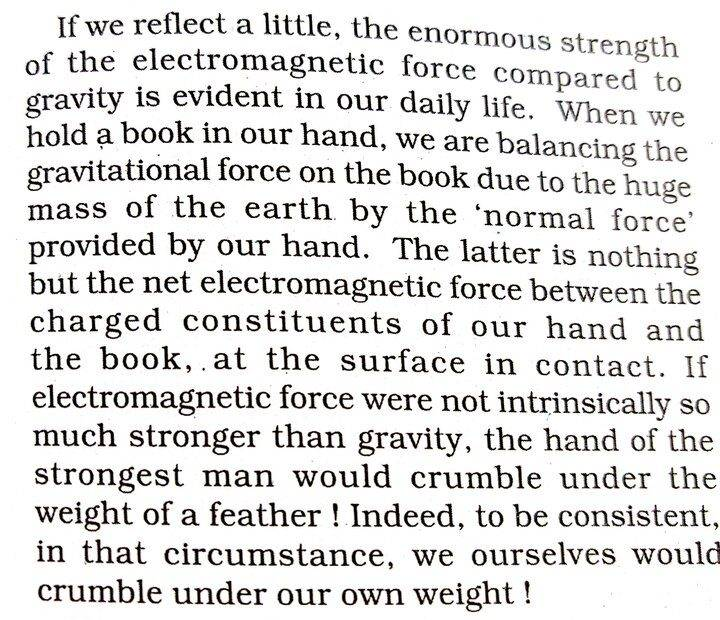# How can the force of a normal reaction be electromagnetic?

• vcsharp2003
In summary, the electromagnetic force between two electrons or protons is much stronger than the gravitational force between them, as shown by the equations for both forces. However, the question of why a person does not crumble under their own weight or why a hand does not crumble under the weight of a feather is not solely explained by electromagnetic forces. Quantum effects, such as the Pauli exclusion principle, also play a role in balancing forces and preventing further contraction. The repulsion between electrons on the surface of objects is what causes surface forces, such as the normal force between a book and a hand. While these effects may be negligible in some cases, they must be considered when explaining phenomena such as neutron stars.

#### vcsharp2003

Homework Statement
I came across the following in a high school textbook, which is confusing. Could someone please explain how a normal contact force is electromagnetic in nature and why our hand would crumble under the weight of a feather if it were not for electromagnetic forces?
Relevant Equations
##F= \frac {Gm_1m_2} {r^2}##
##F= \frac {kq_1q_2} {r^2}##
I do understand that gravitational the electromagnetic force between two electrons or protons is very large compared to the gravitational force between them. I can see this by looking at the equation of gravitational force (##F= \frac {Gm_1m_2} {r^2}##) and the equation of electrical force given by Coulomb's law (##F= \frac {kq_1q_2} {r^2}##).
But I cannot explain why a person would crumble under his or her weight, or a hand would crumble under a feather's weight.

[Mentor Note -- Fixed typo: gravitational --> electromagnetic in the paragraph above]Last edited by a moderator:
Consider two hydrogen atoms, you can not squeeze them arbitrary close together in the hydrogen molecule.
However, it is not entirely due to the electromagnetic force, quantum effects also come into play here.

•topsquark, kuruman and vcsharp2003
malawi_glenn said:
Consider two hydrogen atoms, you can not squeeze them arbitrary close together in the hydrogen molecule.
However, it is not entirely due to the electromagnetic force, quantum effects also come into play here.
The only way a normal force between a book and the holding hand would be electromagnetic in nature is if there are like charges on the surface of the book touching the hand and palm holding the book.

Is this a correct way of explaining it?

vcsharp2003 said:
The only way a normal force between a book and the holding hand would be electromagnetic in nature is if there are like charges on the surface of the book touching the hand and palm holding the book.

Is this a correct way of looking of explaining it?
As some kind of zeroth order explanation yes. Remember that there are plenty of charges in atoms.

It could be interesting for you to know about White Dwarf stars. There the force that balances the gravitational force and prevents further contraction of the stellar remnant is due to a quantum mechanical effect called "Pauli Exclusion principle". Half-integer spin particles (such as electrons) can not occupy the same quantum state and thus exhert an outward pressure.

You can also think about an object, such as piece of metal, why isn't it just collapsing due to gravity? Similar effect as the hydrogen molecule. There is some attraction, but also repulusion. http://hyperphysics.phy-astr.gsu.edu/hbase/molecule/hmol.html

•topsquark and vcsharp2003
Let's simplify this a bit to better understand it. Consider your hand as it pushes a block across a horizontal surface. Why doesn't your hand go through the block? Your hand is made up of molecules, which are made up of atoms. Virtually all the mass of an atom is in the nucleus, which is so tiny compared to the size of the atom that two objects could easily pass through each other. The reason they don't is due to the electromagnetic force holding the atom and molecules together. So when you push on the block the electrons that are on the surface of your hand (bound to the atoms of your hand), encounter the electrons that are on the surface of the block (bound to the atoms of the block). The electrons repulse each other (i.e. push each other away). So as you move your hand, your hand's electrons push against the block's electrons, which push the entire block away.

All surface forces are due to the fact that electrons repeal each other.

So now consider your hand with a block resting on top of it. Why doesn't gravity pull the block through your hand? For the same reason, the electrons of your hand repeal the electrons of the block.

The quantum mechanical effects can be ignored for these cases as they are tiny. But they would have to be included for the case of the neutron star.

•vcsharp2003
should be "electrostatic" (followed by Pauli exclusion principle).

The four fundamental forces are electromagnetic, gravity, weak interaction, and strong interaction. Electrostatic is just a special case of electromagnetic.

•vcsharp2003
Scottpm said:
The quantum mechanical effects can be ignored for these cases as they are tiny.
Are you sure?
If we model an atom as an isolated charge +q at the centre of a conducting shell with a charge -q, would two such repel? I believe the charges on the shell would redistribute so that the net force could be attractive. (Even if we omit the central +q, it turns out there are conditions in which two like-charged conductors will attract.) The atoms would certainly attract until the electron shells are very close.
I would think we need quantum mechanical constraints to prohibit the two shells from becoming well enmeshed.

•vcsharp2003 and topsquark